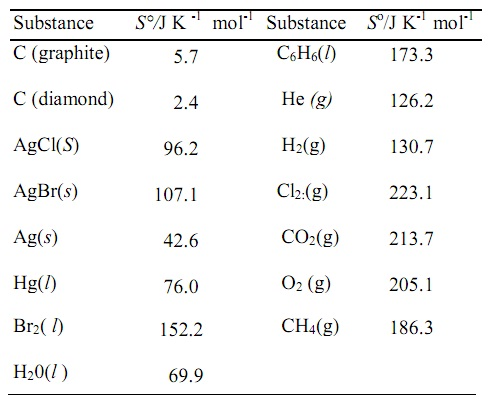#### Entropy of Mixing, Chemistry tutorial

Entropy of mixing:

Assume that that n1 mol of an ideal gas initially present at pressure p and n2 mol of the other ideal gas as well at the similar initial pressure p are mixed at constant temperature in such a way that the total pressure is as well p. This is possible by employing a vessel of appropriate volume. Assume the partial pressure of the first gas in the mixture is p1 and the partial pressure of the other gas is p2. Then, the change in entropy for the first gas from equation ΔS = 2.303 nR log P1/P2 is,

ΔS1 = n1R ln (p/p1)

Likewise, for the other gas,

ΔS2 = n2R ln (p/p2)

The net entropy of mixing, ΔSmix, is therefore

ΔSmix = ΔS1 + ΔS2 = n1R ln p/p1 + n2R ln p/p2

Whenever the mole fractions of the gases in the mixture are x1 and x2 correspondingly, then according to the Dalton's law of partial pressures,

ΔSmix = n1R ln p/x1p + n2R ln p/x2

ΔSmix = n1R ln p/x1p + n2R ln 1/x2

ΔSmix = 2.303 n1R log (l/x1) + 2.303 n2 R log (l/x2)

Therefore,

ΔSmix = 2.303 R[n1 log(1/x1) + n2 log (1/x2)]

ΔSmix = - 2.303 R [n1 log x1 + n2 log x2]

If we are familiar with n1 and n2, ΔSmix can be computed. The mole fractions x1 and x2 are less than one, as x1 + x2 = 1; as an outcome of this, log 1/x1 and log l/x2 are positive. Therefore, ΔSmix is positive.

Entropy changes in Phase transitions:

The change of matter from one phase or stage (that is, solid, liquid, gas, allotropic form) into the other is termed as phase transition. Such changes occur at definite temperatures known as transition temperatures (that is, melting points, boiling points and so on) at a given pressure. Such transitions are accompanied via absorption or evolution of heat (known as latent heat). As absorption or evolution of heat at constant temperature leads to the entropy change, the entropy of transition is represented as,

ΔStrans = qrev/T = ΔHtrans/T

The above equation is valid only if the transition occurs in a reversible manner, that is, if the two phases are in equilibrium.

Therefore, whenever one mole of a solid melts to the liquid phase, the entropy of fusion is represented by:

ΔSfus = ΔHfus/Tf

Here, Tf is the melting point and ΔHfus is the molar enthalpy of fusion. Likewise,

ΔSvap = ΔHvap/TB

Here, TB is the boiling point and ΔHvap is the molar enthalpy of vaporization

It is evident that the entropy of freezing and condensation (that is, vapor into liquid) will be equivalent to - ΔSfus and - ΔSvap, correspondingly.

In a similar way, we can define the entropy change accompanying the transition of a substance from an allotropic form to the other. If such a transition occurs at a temperature Ttrans and ΔHtrans is the molar enthalpy of transition, then the entropy change accompany the transition is,

ΔStrans = ΔHtrans/Ttrans

Therefore, in phase transitions, ΔS values can be computed from the corresponding ΔH values.

Entropy changes in Chemical reactions:

Let us now compute the entropy change accompanying a general chemical reaction of the kind,

aA + bB+....→+ cC + dD + ...

We state the entropy change for a reaction (ΔrS) as the difference between the net entropy of the products and the total entropy of the reactants. Therefore, if SA, SB.... is the entropies of one mole of reactants, A, B and so on, and SC, SD...., of the products, C, D and so on, then;

ΔrS = (cSC + dSD + ....) - (aSA + bSB + .....)

Here c, d, a, b... and so on are the stoichiometric coefficients. The variation of entropy change for a reaction by temperature can be readily deduced from the equation above by differentiating with respect to temperature at constant pressure. Therefore,

[∂(ΔrS)/∂T]p = [c(∂SC/∂T)p + d(∂SD/∂T)p + .....] - [a(∂SA/∂T)p + b (∂SB/∂T)p + ....]

According to the equation:

dH = TdS + Vdp

Or CP‾dT = TdS + Vdp

At constant pressure (dp = 0),

CP‾dT = TdSp

Or dSp/dT = CP‾/T

Or (∂S/∂T) = CP‾/T

CP‾ is the molar heat capacity of the substance at constant pressure.

By utilizing the above result, we obtain:

[∂(ΔrS)/∂T]p = [{cCP‾(C) + dCP‾(D) + ....} - {aCP‾(A) + bCP‾(B) + ....}]/T

[∂(ΔrS)/∂T]p = ΔCp/T

Here, ΔCP is the difference between the heat capacities of the products and reactants at constant pressure.

Or d(ΔrS) = ΔCp (dT/T)

Suppose that ΔrS1 and ΔrS2 are the entropy change at temperatures T1 and T2 and ΔCp is independent of the temperature. Then the above equation on integration gives,

ΔrS1ΔrS2 d(ΔrS) = ΔCp T1T2 (dT/T)

Or ΔrS2 - ΔrS1 = Cp ln T2/T1

The above equation is helpful in finding out ΔrS value of a reaction at any specific temperature, if it is recognized at any other temperature all along by Cp values.

The entropy values of substances can be found out by using the third law of thermodynamics. The entropy values of some of the substances in their standard states at 298.15 K are represented in the table. These are termed as standard entropy (S°) values. Similar to the computation of AJS, we can compute ΔrS° from the standard entropy values of the reactants and the products.

Table: Standard Entropy (S°) values at 298.15 KTutorsglobe: A way to secure high grade in your curriculum (Online Tutoring)